Search USP29

GENERAL CONSIDERATIONS
Fundamental Decay Law
The decay of a radioactive source is described by the equation:
in which Nt is the number of atoms of a radioactive substance at elapsed time t, No is the number of those atoms when t = 0, and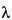is the transformation or decay constant, which has a characteristic value for each radionuclide. The half-life, T1/2, is the time interval required for a given activity of a radionuclide to decay to one-half of its initial value, and is related to the decay constant by the equation:
The activity of a radioactive source (A) is related to the number of radioactive atoms present by the equation:
from which the number of radioactive atoms at time t can be computed, and hence the mass of the radioactive material can be determined.
The activity of a pure radioactive substance as a function of time can be obtained from the exponential equation or from decay tables, or by graphical means based on the half-life (see Normalized Decay Chart, Figure 1).
Fig. 1. Normalized Decay Chart.
The activity of a radioactive material is expressed as the number of nuclear transformations per unit time. The fundamental unit of radioactivity, the curie (Ci), is defined as 3.700 × 1010 nuclear transformations per second. The millicurie (mCi) and microcurie (µCi) are commonly used subunits. The “number of nuclear transformations per unit time” is the sum of rates of decay from all competing modes of disintegration of the parent nuclide. Before the activity of any given radionuclide in a measured specimen can be expressed in curies, it is often necessary to know the abundance(s) of the emitted radiation(s) measured.
Geometry
The validity of relative calibration and measurement of radionuclides is dependent upon the reproducibility of the relationship of the source to the detector and its surroundings. Appropriate allowance must be made for source configuration.
Background
Cosmic rays, radioactivity present in the detector and shielding materials, and radiation from nearby radioactive sources not properly shielded from the measuring equipment, all contribute to the background count rate. All radioactivity measurements must be corrected by subtracting the background count rate from the gross count rate in the test specimen.
Statistics of Counting
Since the process of radioactive decay is a random phenomenon, the events being counted form a random sequence in time. Therefore, counting for any finite time can yield only an estimate of the true counting rate. The precision of this estimate, being subject to statistical fluctuations, is dependent upon the number of counts accumulated in a given measurement and can be expressed in terms of the standard deviation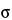. An estimate foris
where n is the number of counts accumulated in a given measurement. The probability of a single measurement falling within
of the mean of a great many measurements is 0.68. That is, if many measurements of n counts each were to be made, approximately two-thirds of the observations would lie within
of the mean, and the remainder outside.
Because of the statistical nature of radioactive decay, repeated counting of an undisturbed source in a counting assembly will yield count-rate values in accordance with the frequency of a normal distribution. Deviations in these values from the normal distribution conform to the2 test. For this reason, the2 test is frequently applied to determine the performance and correct operation of a counting assembly. In the selection of instruments and conditions for assay of radioactive sources, the figure of merit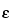2/B should be maximized (where= counter efficiency = observed count rate/sample disintegration rate, and B = background count rate).
Counting Losses
The minimum time interval that is required for the counter to resolve two consecutive signal pulses is known as the dead time. The dead time varies typically from the order of microseconds for proportional and scintillation counters, to hundreds of microseconds for Geiger-Müller counters. Nuclear events occurring within the dead time of the counter will not be registered. To obtain the corrected count rate, R, from the observed count rate, r, it is necessary to use the formula:
in which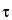is the dead time. The foregoing correction formula assumes a nonextendable dead time. Thus, for general validity, the value of rshould not exceed 0.1. The observed count rate, r, refers to the gross specimen count rate and is not to be corrected for background before use in the foregoing equation.
Calibration Standards
Perform all radioactivity assays using measurement systems calibrated with appropriately certified radioactivity standards. Such calibration standards may be purchased either direct from the National Institute of Standards and Technology or from other sources that have established traceability to the National Institute of Standards and Technology through participation in a program of inter-comparative measurements. Where such calibration standards are unavailable, the Pharmacopeia provides the nuclear decay data required for calibration. These data, as well as half-life values, are obtained from the Evaluated Nuclear Structure Data File of the Oak Ridge Nuclear Data Project, and reflect the most recent values at the time of publication.
Carrier
The total mass of radioactive atoms or molecules in any given radioactive source is directly proportional to the activity of the radionuclide for a given half-life, and the amount present in radiopharmaceuticals is usually too small to be measured by ordinary chemical or physical methods. For example, the mass of 131I having an activity of 100 mCi is 8 × 10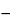7 g. Since such small amounts of material behave chemically in an anomalous manner, carriers in the form of nonradioactive isotopes of the same radionuclide may be added during processing to permit ready handling. In many cases, adsorption can be prevented merely by increasing the hydrogen-ion concentration of the solution. Amounts of such material, however, must be sufficiently small that undesirable physiological effects are not produced. The term “carrier-free” refers only to radioactive preparations in which nonradioactive isotopes of the radionuclide are absent. This implies that radioactive pharmaceuticals produced by means of (n,) reactions cannot be considered carrier-free.
The activity per unit volume or weight of a medium or vehicle containing a radionuclide either in the carrier-free state or in the presence of carrier is referred to as the radioactive concentration, whereas the term specific activity is used to express the activity of a radionuclide per gram of its element.
Individual radiopharmaceutical monographs indicate the expiration date, the calibration date, and the statement, “Caution—Radioactive Material.” The labeling indicates that in making dosage calculations, correction is to be made for radioactive decay, and also indicates the radioactive half-life of the radionuclide. Articles that are Injections comply with the requirements for Labeling under Injections1, and those that are Biologics comply with the requirements for Labeling under Biologics1041.